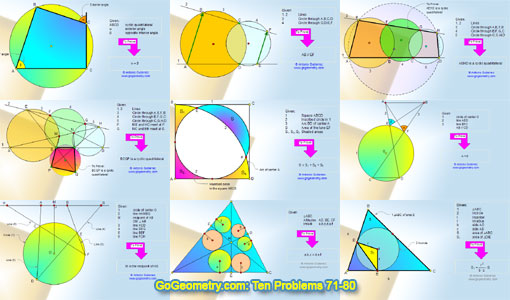Ten Geometry Problems 71-80. Level: High School, CollegeGeometry Problems 71 - 80Angles.

Proposed Problem 72: Intersecting Circles.

Proposed Problem 73: Three Intersecting Circles. Cyclic quadrilateral.

Proposed Problem 74: Three Intersecting Circles. Cyclic quadrilateral, Angles.

Proposed Problem 75: Three Intersecting Circles. Cyclic quadrilateral, Angles.
Acquire and demonstrate mathematical reasoning ability when solving problems.

Proposed Problem 76: Area of a Circle. Square, Circle, Circular Sector.

Proposed Problem 77: Angles of a Circle. Parallel lines, Cyclic quadrilateral.

Proposed Problem 78: Angles of a Circle. Perpendicular and parallel lines, Midpoint, Diameter, Chord, Cyclic quadrilateral, Congruence.

Proposed Problem 79: Triangle. Similarity, Altitudes, Orthocenter, Incircles, Inradii.

Proposed Problem 80. Area of a triangle, side, incircle, inradius.

Poster of problem 80. Area of a triangle, side, incircle, inradius.

Home | Sitemap | Search | Geometry | Problems | Visual Index | 10 ProblemsEmail | Post a comment | by Antonio Gutierrez
May 28, 2018